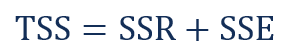# Sum of Squares

A statistical tool that is used to identify the dispersion of data

## What is Sum of Squares?

Sum of squares (SS) is a statistical tool that is used to identify the dispersion of data as well as how well the data can fit the model in regression analysis. The sum of squares got its name because they are calculated by finding the sum of the squared differences.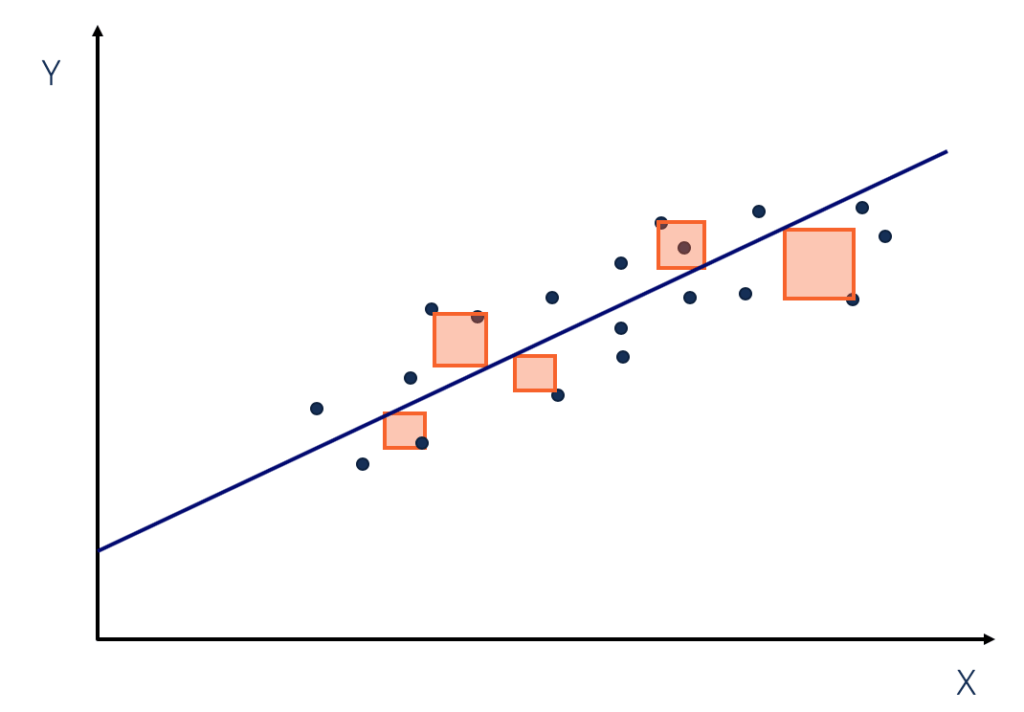This image is only for illustrative purposes.

The sum of squares is one of the most important outputs in regression analysis. The general rule is that a smaller sum of squares indicates a better model as there is less variation in the data.

In finance, understanding the sum of squares is important because linear regression models are widely used in both theoretical and practical finance.

### Types of Sum of Squares

In regression analysis, the three main types of sum of squares are total sum of squares, regression sum of squares, and residual sum of squares.

#### 1. Total sum of squares

The total sum of squares is a variation of the values of a dependent variable from the sample mean of the dependent variable. Essentially, the total sum of squares quantifies the total variation in a sample. It can be determined using the following formula: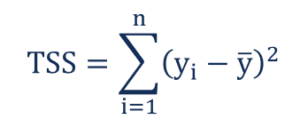Where:

• y– the value in a sample
• ȳ – the mean value of a sample

#### 2. Regression sum of squares (also known as sum of squares due to regression or explained sum of squares)

The regression sum of squares describes how well a regression model represents the modeled data. The regression type of sum of squares indicates how well the regression model explains the data. A higher regression sum of squares indicates that the model does not fit the data well.

The formula for calculating the regression sum of squares is: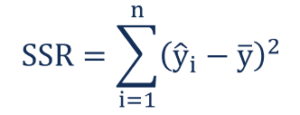Where:

• ŷ– the value estimated by the regression line
• ȳ – the mean value of a sample

#### 3. Residual sum of squares (also known as sum of squared errors of prediction)

The residual sum of squares essentially measures the variation of modeling errors. In other words, it depicts how the variation in the dependent variable in a regression model cannot be explained by the model. Generally, a lower residual sum of squares indicates that the regression model can better explain the data while a higher residual sum of squares indicates that the model poorly explains the data.

The residual sum of squares can be found using the formula below: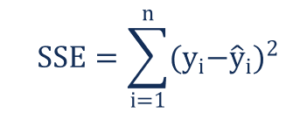Where:

• y– the observed value
• ŷ– the value estimated by the regression line

The relationship between the three types of sum of squares can be summarized by the following equation: